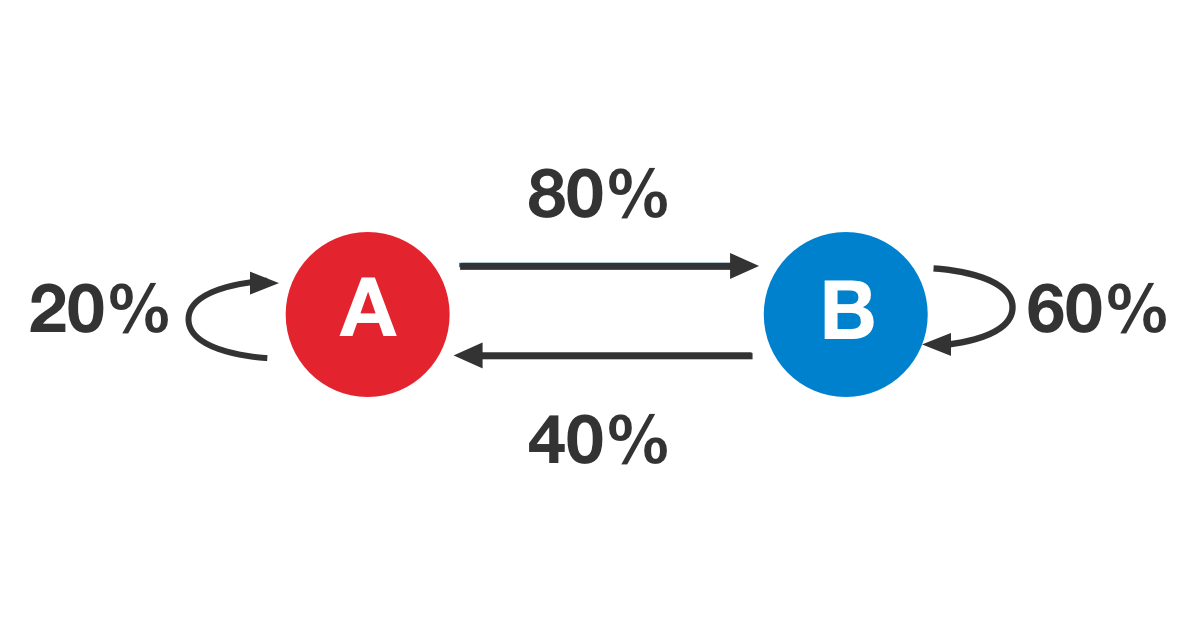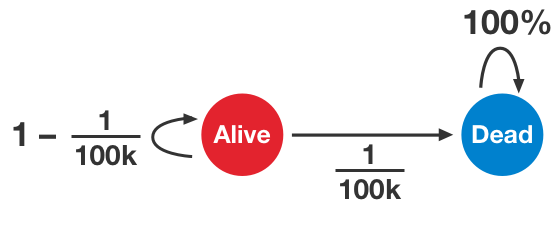Probability

# Basic Markov Chains

In the Markov chain pictured, what is the probability that a process beginning at state A will be at state B after $2$ moves?Let $k$ represent the number of days until a person turns $200$ years old. What is the biggest problem with this model of life?

Any general stochastic process can be made to satisfy the Markov property by altering the state space (and adding probabilities for any new states). In this way, it can "turn into" a Markov chain. Given a stochastic process with state space $S$, which of the following methods would create a Markov chain that models the same process?

1. Alter $S$ to instead contain sequences of states, the $k^\text{th}$ element of which tells where the Markov chain was at step $k$.
2. Change $S$ to contain pairs of states $(i, \, j)$ instead. That way, the Markov chain can know both the present state and the previous state.
3. Modify $S$ to contain only one state, but make its transition probabilities contain all the information about the stochastic process.
4. Don't change $S$, but make the transition probabilities satisfy the Markov property.
5. Add a large number of "in-between" states to $S$, to create a bunch of little steps satisfying the Markov property.

The Golden State Warriors are strongly affected by morale, and they win games according to the probabilities in the following table:

 States Win Tomorrow Lose Tomorrow Won Today $\tfrac{4}{5}$ $\tfrac{1}{5}$ Lost Today $\tfrac{1}{3}$ $\tfrac{2}{3}$

If they lose the first two games in a "best of $7$" (first to $4$) series, what is the probability that they will be able to come back and win it?In the Markov chain pictured, what is the probability that a process beginning at state A will be back at state A after $3$ moves?

×